Armands Gritsans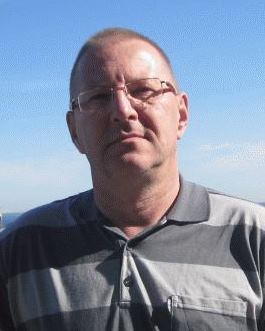Leading researcher, associate professor, Dr.math. Mathematical Research Center Department of Technology Institute of Life Sciences and Technology Daugavpils University Parades street 1 LV-5400, Daugavpils, Latvia armands.gricans@du.lv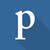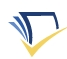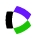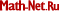Research interests Nonlinear boundary problems for ordinary differential equations (asymmetric problems, qualitative behavior of solutions of nonlinear differential equations), discrete dynamical systems, differential-geometric structures on manifolds Education Dr.math., University of Latvia, 1992.Ph.D. (Candidate of Physico-Mathematical Sciences), Moscow State Pedagogical University, 1991,  thesis: "Differential geometry of Killing f-structures on manifolds" (summary and link to the summary). Advisor: prof. V. Kirichenko. Teaching Academic bachelor study programme "Mathematics": mathematical software, graph theory, mathematics history, functional analysis, Lebesgue measure and integral, theory of functions of a complex variable Academic master study programme "Mathematics": mathematical software, discrete dynamical systems, discrete mathematics Doctoral study programme "Mathematics": mathematical software Selected publications A. Gritsans and , On the maximum number of period annuli for second order conservative equations, Vol. 26, No. 4,  2021, pp. 612-630. A. Gritsans, V. Koliskina, A. Kolyshkin, and , On the stability of a steady convective flow in a vertical layer of a chemically reacting fluid, in: 9th edition of the International Conference on Computational Methods for Coupled Problems in Science and Engineering (COUPLED PROBLEMS 2021), Vol. IS34 - Multiphysics Problems, 2021, 10 pp. A. Gritsans, A. Kolyshkin, D. Ogorelova, , I. Samuilik, and , Solutions of nonlinear boundary value problem with applications to biomass thermal conversion, Proceedings of 20th International Scientific Conference "Engineering for rural development" (May 26-28, Jelgava, Latvia), 2021, pp. 837-842. A. Gritsans, Asymptotically stable heteroclinic cycles in discrete-time Z4-equivariant cubic dynamical systems, Journal of Difference Equations and Applications, Vol. 26, Issue 9-10, 2020, pp. 1247-1265. A. Gritsans and , A two-point boundary value problem for third order asymptotically linear systems, Electronic Journal of Qualitative Theory of Differential Equations, No. 28, 2019, 24 pp. A. Gritsans, On a one-parameter discrete-time Z4-equivariant cubic dynamical system in Applied Sciences and Engineering, Vol. 29, No. 4, 1950052 (2019), 15 pp. A. Gritsans, F. Sadyrbaev, and I. Yermachenko, Dirichlet boundary value problem for a system of n second order asymptotically asymmetric differential equations, Electronic Journal of Differential Equations, Vol. 2018 (2018), No. 35, pp. 1-16. A. Gritsans, F. Sadyrbaev, and I. Yermachenko, Dirichlet boundary value problem for the second order asymptotically linear system.  (2016), Article ID 5676217, 12 pp. A. Gritsans and F. Sadyrbaev, The Nehari solutions and asymmetric minimizers, Discrete Contin. Dyn. Syst. 2015, Dynamical systems, differential equations and applications, 10th AIMS Conference. Suppl., 2015, 562-568. A. Gritsans and F. Sadyrbaev, Extension of the example by Moore–Nehari,  Vol. 63, No. 2.,  2015, 115-127. A. Gritsans and F. Sadyrbaev, Unexpected solutions of the Nehari problem, International Journal of Analysis, Vol. 2014 (2014), Article ID 467831, 5 pages. A. Gritsans and F. Sadyrbaev, On solvability of boundary value problem for asymmetric differential equation depending on x',  Vol. 18, No. 2,  2013, 176-190. A. Gritsans and F. Sadyrbaev, Boundary value problems for a super-sublinear asymmetric oscillator: exact number of solutions, International Journal of Mathematics and Mathematical Sciences, Vol. 2013 (2013), Article ID 705984, 9 pages. A. Gritsans and , On Fučik type spectra, Proceedings of the Institute of Mathematics and Computer Science of the University of Latvia. Mathematics. Differential equations, Vol. 12, 2012, pp. 75–84. A. Gritsans and F. Sadyrbaev, Nonlinear problems with asymmetric principal part, Vol. 17, No. 2,  2012, 217-226. A. Gritsans and F. Sadyrbaev, Two-parameter nonlinear oscillations: the Neumann problem, Vol. 16, No. 1,  2011, 23-38. A. Gritsans and , Properties of a nonlinear asymmetric oscillator with description of spectra, Proceedings of the Institute of Mathematics and Computer Science of the University of Latvia. Mathematics. Differential equations, Vol. 11, 2011, pp. 90–101. A. Gritsans and , On solution set of a two-parameter nonlinear oscillator: the Neumann problem, Proceedings of the Institute of Mathematics and Computer Science of the University of Latvia. Mathematics. Differential equations, Vol. 9/10, 2010, pp. 66–76. A. Gritsans, F. Sadyrbaev, and N. Sergejeva, Two parameter nonlinear eigenvalue problems, Mathematical Models in Engineering, Biology, and Medicine, Proceedings of the International Conference on Boundary Value Problems2009, Vol. 1124, 185-194. A. Gritsans and  Nonlinear spectra: the Neumann problem, Mathematical Modelling and Analysis, Vol. 14, No. 1,  2009, 33-42. A. Gritsans and , On nonlinear Fučik type spectra, Mathematical Modelling and Analysis, Vol. 13, No. 2,  2008, 203-210. A. Gritsans and F. Sadyrbaev, Two-parametric nonlinear eigenvalue problems. Electronic Journal of Qualitative Theory of Differential Equations, Proc. 8'th Coll. Qualitative Theory of Diff. Equ., No. 10, 2008, 1-14. A. Gritsans and , Time map formulae and their applications, Proceedings of the Institute of Mathematics and Computer Science of the University of Latvia. Mathematics. Differential equations, Vol. 8, 2008, pp. 72–93. and A. Gritsans, Nonlinear spectra for parameter dependent ordinary differential equations. Nonlinear Analysis: Modelling and Control, Vol. 12, No. 2, 2007, 253–267. A. Gritsans and F. Sadyrbaev, On the Nehari solutions, Proceedings of Equadiff 11, International Conference on Differential Equations. Czecho-Slovak series, Bratislava, July 25-29, 2005. [Part 2] Minisymposia and contributed talks. Comenius University Press, Bratislava, 2007, pp. 437-446. A. Gritsans and , Nonlinear spectra for two-parameter eigenvalue problems, Proceedings of the Institute of Mathematics and Computer Science of the University of Latvia. Mathematics. Differential equations, Vol. 7, 2007, pp. 71–94. A. Gritsans and Characteristic numbers of non-autonomous Emden-Fowler type equations, Mathematical Modelling and Analysis, Vol. 11, No. 3,  2006, 243-252. A. Gritsans and , On nonlinear eigenvalue problems, Proceedings of the Institute of Mathematics and Computer Science of the University of Latvia. Mathematics. Differential equations, Vol. 6, 2006, pp. 76–86. A. Gritsans and F. Sadyrbaev, Characteristic numbers of non-autonomous Emden-Fowler type equations. Proceedings: 10th International Conference "Mathematical Modelling and Analysis 2005'' and 2nd International Conference "Computational Methods in Applied Mathematics'', 403--408, Technika, Vilnius, 2005. A. Gritsans and F. Sadyrbaev, Remarks on lemniscatic functions, Acta Universitatis Latviensis, Vol. 688. Mathematics, 2005, 39-50.  pdf dspace.lu.lv A. Gritsans and F. Sadyrbaev, The Taylor series expansion coefficients for solutions of the Emden-Fowler type equations, Mathematical Modelling and Analysis, Vol. 10, No. 3, 2005, 247-256. A. Gritsans and , Explicit solutions of non-autonomous Emden-Fowler type equations, Proceedings of the Institute of Mathematics and Computer Science of the University of Latvia. Mathematics. Differential equations, Vol. 5, 2005, pp. 5–23. A. Gritsans and , Trigonometry of lemniscatic functions, Proceedings of the Institute of Mathematics and Computer Science of the University of Latvia. Mathematics. Differential equations, Vol. 4, 2004, pp. 22–29. A. Gritsans and , Lemniscatic functions in the theory of the Emden-Fowler differential equation, Proceedings of the Institute of Mathematics and omputer Science of the University of Latvia. Mathematics. Differential equations, Vol. 3, 2003, pp. 5–27. А.С. Грицанс, О строении киллинговых f-многообразий, Изв. вузов. Матем., 1992, No. 6, 46–54.A.S. Gritsans, On construction of Killing f-manifolds, Izv. Vyssh. Uchebn. Zaved. Mat., No. 6, 1992, 46–54.А.С. Грицанс, О геометрии киллинговых f-многообразий, УМН, 1990, том 45, выпуск 4(274), 149–150.A.S. Gritsans, On the geometry of Killing f-manifolds, Russ. Math. Surv., Vol. 45, No. 4, 1990, 168-169. IOPscience A.S. Gritsans,  Some distributions on Killing f-manifolds (Russian), Webs and quasigroups, pp. 142-146, Kalinin. Gos. Univ., Kalinin, 1990. A.S. Gritsans,  On a hyperspherical mapping and the Peterson transformation of the surface Vp\subset En by means of the medial normal basis vector (Russian), Mathematics, I, pp. 152-161, Latv. Univ. Zināt. Raksti, 552, Latv. Univ., Riga, 1990. A.S. Gritsans,  On the geometry of a family of middle normals of a surface Vp\subset En (Russian), Differentsial'naya Geom. Mnogoobraz. Figur, No. 20, 1989, pp. 34-37. A.S. Gritsans, p-surfaces in En with a general family of mean normals (Russian), Differentsial'naya Geom. Mnogoobraz. Figur, No. 18, 1987, p. 25-27. A.S. Gritsans, On the family of middle normals of the surface V2\subset E5 (Russian), Differentsial'naya Geom. Mnogoobraz. Figur, No. 17, 1986, pp. 21-25. A.S. Gritsans, The differential equation dx/dt=Ax in a Banach space (Russian), Continuous functions on topological spaces, pp. 64-68, iii, xi, Latv. Gos. Univ., Riga, 1986. Activities DU project coordinator of Fundamental and applied research project of the Latvian Council of Science "Analysis of complex dynamical systems in fluid mechanics and heat transfer", lzp-2021/1-0076 Researcher of Fundamental and applied research project of the Latvian Council of Science "Bryophyte and lichen successional and spatial patterns in deciduous forests", lzp-2020/1-0314 Editorial Board Member, The Baltic Journal on Mathematical Applications, Numerical Analysis and Differential Equations "Mathematical Modelling and Analysis" Member of the Latvian Mathematical Society Member of the International Society of Difference Equations Chairman of the Organizing Committee of the 14th International Conference "Mathematical Modelling and Analysis", May 27 - 30, 2009, Daugavpils, Latvia Leader of the European Social Fund project "The modernization of the informative and technical equipment of mathematics and its applications studies in the Daugavpils University"  2006/0245/VPD1/ESF/PIAA/06/APK/3.2.3.2./0053/0065 (01.01.2007.-31.12.2007.)